## NCERT Solutions for Class 7 Science Chapter 11 Transportation in Animals and Plants

NCERT Solutions for Class 7 Science Chapter 11 Transportation in Animals and Plants are part of NCERT Solutions for Class 7 Science. Here we have given NCERT Solutions for Class 7 Science Chapter 11 Transportation in Animals and Plants.

 Board CBSE Textbook NCERT Class Class 7 Subject Science Chapter Chapter 11 Chapter Name Transportation in Animals and Plants Number of Questions Solved 13 Category NCERT Solutions

## NCERT Solutions for Class 7 Science Chapter 11 Transportation in Animals and Plants

Question 1.
Match structures given in Column I with functions given in Column II:

 Column I Cotumn II (i) Stomata (a) Absorption of water (ii) Xylem (b) Transpiration (iii) Root hairs (c) Transport of food (iv) Phloem (d) Transport of water (e) Synthesis of carbohydrates

 Column I Cotumn II (i) Stomata (b) Transpiration (ii) Xylem (d) Transport of water (iii) Root hairs (a) Absorption of water (iv) Phloem (c) Transport of food

Question 2.
Fill in the blanks:

1. The blood from the heart is transported to all parts of the body by the ………..
2. Hemoglobin is present in cells.
3. Arteries and veins are joined by a network of ……….
4. The rhythmic expansion and contraction of the heart is called …………
5. The main excretory product in human beings is ………..
6. Sweat contains water and ……….
7. Kidneys eliminate the waste materials in the liquid form called ………..
8. Water reaches great heights in the trees because of suction pull caused by ………..

1. arteries
2. red blood
3. capillaries
4. heartbeat
5. urea
6. salts
7. urine
8. transpiration

Question 3.
Choose the correct option:
(a) In plants, water is transported through
(i) xylem
(ii) phloem
(iii) stomata
(iv) root hair

(b) Water absorption through roots can be increased by keeping the plants
(ii) in dim light
(iii) under the fan
(iv) covered with a polythene bag
(a) (i) xylem
(b) (iii) under the fan.

Question 4.
Why is the transport of materials necessary in a plant or in an animal? Explain.
Transport of materials is essential for plants or animals because nutrients and oxygen are made available to all the parts of the body through transpiration. If the transport of necessary nutrients and oxygen does not take place in the body, the body will not be able to survive.

Question 5.
What will happen if there are no platelets in the blood?
Whenever we get injured, blood comes out from the cut. The function of platelets is to make a clot which plugs the cut to stop bleedings. If there are no platelets in the blood, no clot will be formed and as a result, bleeding will not stop and leads to a disease: haemophilia. This may lead to fatal consequences.

Question 6.
What are stomata? Give two functions of stomata.
Stomata are the tiny pores present on the surface of the leaves. These are surrounded by two bean shaped cells called guard cells.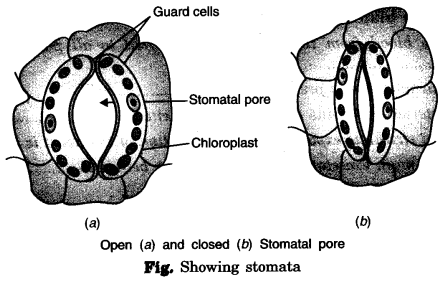Functions of stomata :
(i) The exchange of gases in plants occurs through stomata.
(ii) The excess water in plants evaporates through stomata by a process called transpiration.

Question 7.
Does transpiration serve any useful function in the plants? Explain.
Due to transpiration, a suction pressure develops which helps in the transportation of water.

Question 8.
What are the components of blood?
Blood has two components: (a) Plasma and (b) Corpuscles, suspended in the plasma, with three kinds of blood cells.
(а) Plasma: It is the fluid part of blood.
(b) Corpuscles: It is of three types:
(i) Red Blood Cells (RBCs): Contain hemoglobin and impart a red color to the blood. Haemoglobin also carries oxygen from the lungs to all other body parts.
(ii) White Blood Cells (WBCs): Fight against the germs.
(iii) Platelets: Help in the clotting of blood.

Question 9.
Why is blood needed by all the parts of a body?
Blood carries digested food and oxygen to all parts of the body. It also carries away the waste products.

Question 10.
What makes the blood look red?
Blood contains three kinds of blood cells. Out of which Red Blood Cells contain an iron pigment called haemoglobin. Hemoglobin imparts red colour to the blood.

Question 11.
Describe the function of the heart.
The heart collects and distributes purified blood to all parts of the body through arteries.

Question 12.
Why is it necessary to excrete waste products?
Waste products are harmful to the body.

Question 13.
Draw a diagram of the human excretory system and label the various parts.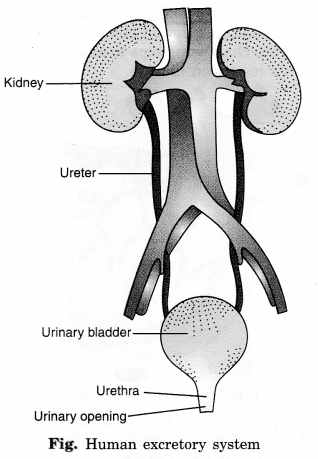We hope the NCERT Solutions for Class 7 Science Chapter 11 Transportation in Animals and Plants help you. If you have any query regarding NCERT Solutions for Class 7 Science Chapter 11 Transportation in Animals and Plants, drop a comment below and we will get back to you at the earliest.

## NCERT Solutions for Class 7 Maths Chapter 5 Lines and Angles Ex 5.1

NCERT Solutions for Class 7 Maths Chapter 5 Lines and Angles Ex 5.1 are part of NCERT Solutions for Class 7 Maths. Here we have given NCERT Solutions for Class 7 Maths Chapter 5 Lines and Angles Ex 5.1.

 Board CBSE Textbook NCERT Class Class 7 Subject Maths Chapter Chapter 5 Chapter Name Lines and Angles Exercise Ex 5.1, Ex 5.2. Number of Questions Solved 14 Category NCERT Solutions

## NCERT Solutions for Class 7 Maths Chapter 5 Lines and Angles Ex 5.1

Question 1.
Find the complement of each of the following angles:Solution:
Since, the sum of the measures of an angle and its complement is 90°, therefore,

1. The complement of an angle of measure 20° is the angle of (90° – 20°), f.e., 70°.
2. The complement of an angle of measure 63° is the angle of (90° – 63°), i.e., 27°.
3. The complement of an angle of measure 57° is the angle of (90° – 57°), i.e., 33°.

Question 2.
Find the supplement of each of the following angles:Solution:

1. Supplement of the angle 105° = 180° – 105° = 75°
2. Supplement of the angle 87° = 180° – 87° = 93°
3. Supplement of the angle 154° = 180° – 154° = 26°

Question 3.
Identify which of the following pairs of angles are complementary and which are supplementary.

1. 65°, 115°
2. 63°, 27°
3. 112°, 68°
4. 130°, 50°
5. 45°,45°
6. 80°, 10°.

Solution:

1. Since, 65°+ 115° = 180°
So, this pair of angles are supplementary.
2. Since, 63°+ 27° = 90°
So, this pair of angles are complementary.
3. Since, 112° + 68° = 180°
So, this pair of angles are supplementary.
4. Since, 130°+50° = 180°
So, this pair of angles are supplementary.
5. Since, 45°+ 45° = 90°
So, this pair of angles are complementary.
6. Since, 80°+ 10° = 90°
So, this pair of angles are complementary.

Question 4.
Find the angle which is equal to its complement.
Solution:
Let the measure of the angle be x°. Then, the measure of its complement is given to be x°.
Since, the sum of the measures of an angle and its complement is 90°, therefore,
x° + x° = 90°
⇒ 2x° = 90°
⇒ x° = 45°
Thus, the required angle is 45°.

Question 5.
Find the angle which is equal to its supplement.
Solution:
Let the measure of the angle be x°. Then,
a measure of its supplement = x°
Since, the sum of the measures of an angle and its supplement is 180°, therefore,
x° + x° = 180°
⇒ 2x° =180°
⇒ x° = 90°
Hence, the required angle is 90°.

Question 6.
In the given figure, ∠ 1 and ∠ 2 are supplementary angles.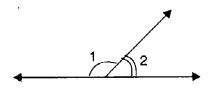If ∠1 is decreased, what changes should take place in ∠ 2 so that both the angles still remain supplementary?
Solution:
∠ 2 will increase as much as ∠ 1 decreases.

Question 7.
Can two angles be supplementary if both of them are:

1. acute?
2. obtuse?
3. right?

Solution:

1. No! two acute angles cannot be a supplement.
2. No! Two obtuse angles cannot be supplementary.
3. Yes! Two right angles are always supplementary.

Question 8.
An angle is greater than 45°. Is its complementary angle greater than 45° or equal to 45° or less than 45°.
Solution:
Since the sum of the measure of ah angle and its complement is 90°.
∴ The complement of an angle of measures 45° + x°,
where x > 0 is the angle of [90° – (45° + x°)] = 90° – 45° – x°= 45° – x°.
Clearly, 45° + x° > 45° – x°
Hence, the complement of an angle > 45° is less than 45°.

Question 9.1. Is ∠1 adjacent to ∠2 ?
2. Is ∠ AOC adjacent to ∠ AOE?
3. Do ∠ COE and ∠ EOD form a linear pair?
4. Are ∠ BOD and ∠ DOA supplementary?
5. Is ∠ 1 vertically opposite to ∠ 4?
6. What is the vertically opposite angle of ∠ 5?

Solution:

1. Yes ! ∠ 1 is adjacent to ∠ 2.
2. No ! ∠ AOC is not adjacent to ∠ AOE.
3. Yes! ∠ COE and ∠ EOD form a linear pair.
4. Yes ! ∠ BOD and ∠ DOA are supplementary.
5. Yes ! ∠ 1 is vertically opposite to ∠ 4.
6. The vertically opposite angle of ∠ 5 is ∠ 2 + ∠ 3, i.e., ∠ COB.

Question 10.
Indicate which pairs of angles are: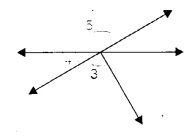1. Vertically opposite angles.
2. Linear pairs.

Solution:

1. The pair of vertically opposite angles are ∠1, ∠4; ∠5, ∠2 + ∠3.
2. The pair of linear angles are ∠1, ∠5; ∠4, ∠5.

Question 11.
In the following figure, is ∠ 1 adjacent to ∠ 2? Give reasons.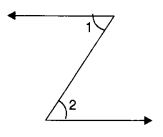Solution:
∠1 is not adjacent to ∠2 because they have no common vertex.

Question 12.
Find the values of the angles x, y, and z in each of the following: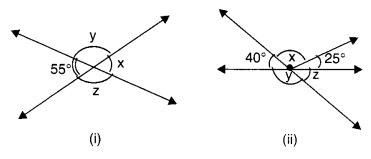Solution: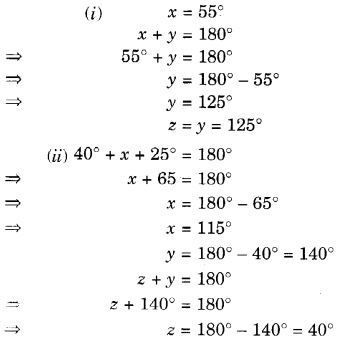Question 13.
Fill in the blanks:

1. If two angles are complementary, then the sum of their measures is
2. If two angles are supplementary, then the sum of their measures is
3. Two angles forming a linear pair are
4. If two adjacent angles are supplementary, they form a
5. If two lines intersect at a point, then the vertically opposite angles are always
6. If two lines intersect at a point, and if one pair of vertically opposite angles are acute angles, then the other pair of vertically opposite angles are

Solution:

1. 90°
2. 180°
3. supplementary
4. linear pair
5. equal
6. obtuse angles

Question 14.
In the adjoining figure, name the following pairs of angles.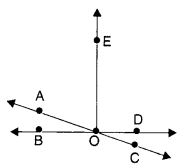1. Obtuse vertically opposite angles
3. Equal supplementary angles
4. Unequal supplementary angles
5. Adjacent angles that do not form a linear pair.

Solution:

1. Obtuse vertically opposite angles are ∠AOD and ∠BOC.
2. Adjacent complementary angles are ∠BOA and ∠AOE.
3. Equal supplementary angles are ∠BOE and ∠EOD.
4. Unequal supplementary angles are ∠BOA and ∠AOD, ∠BOC and ∠COD, ∠EOA, and ∠EOC.
5. Adjacent angles that do not form a linear pair are ∠AOB and ∠AOE, ∠AOE and ∠EOD; ∠EOD and ∠COD.

We hope the NCERT Solutions for Class 7 Maths Chapter 5 Lines and Angles Ex 5.1 help you. If you have any query regarding NCERT Solutions for Class 7 Maths Chapter 5 Lines and Angles Ex 5.1, drop a comment below and we will get back to you at the earliest.

## NCERT Solutions for Class 7 Science Chapter 16 Water: A Precious Resource

NCERT Solutions for Class 7 Science Chapter 16 Water: A Precious Resource are part of NCERT Solutions for Class 7 Science. Here we have given NCERT Solutions for Class 7 Science Chapter 15 Light.

 Board CBSE Textbook NCERT Class Class 7 Subject Science Chapter Chapter 16 Chapter Name Water: A Precious Resource Number of Questions Solved 9 Category NCERT Solutions

## NCERT Solutions for Class 7 Science Chapter 16 Water: A Precious Resource

Question 1.
Mark ‘T if the statement is true and F’ if it is false:
(а) The freshwater stored in the ground is much more than that present in the rivers and lakes of the world. (T/F)
(b) Water shortage is a problem faced only by people living in rural areas. (T/F)
(c) Water from rivers is the only source for irrigation in the fields. (T/F)
(d) Rain is the ultimate source of water. (T/F)
(a) T
(b) F
(c) F
(d) T

Question 2.
Explain how groundwater is recharged?
The rainwater and water from other sources such as rivers and ponds seeps through the soil and fills the empty spaces and cracks deep below the ground. The process of seeping water into the ground is called infiltration. The groundwater thus gets recharged by this process.

Question 3.
There are ten tube wells in a lane of fifty houses. What could be the long-term impact on the water table?
A huge amount of water will be pumped out from these ten tube wells and it will result in the depletion of the groundwater. Consequently, the water table will go deeper and deeper with time and in the longer-term, it will affect the availability of water in that area.

Question 4.
You have been asked to maintain a garden. How will you minimize the use of water?
The use of water can be minimized by:

• using drip irrigation.
• preventing the leakage in the drains in the delivery pipes.
• filling the burrows of snakes and rats.
• protecting the boundaries of the field from breakage.

Question 5.
Explain the factors responsible for the depletion of the water table.
There are three main causes of depletion of the water table.
(i) Increasing population:
The increasing population creates demand for the construction of houses, shops, offices, roads, and pavements. This decreases the open areas like parks and playgrounds. This, in turn, decreases the seepage of rainwater into the ground. Moreover, a huge amount of water is required for construction work. Often groundwater is used for this purpose. So, on the one hand, we are consuming more groundwater whereas, on the other hand, we are allowing lesser water to seep into the ground. This results in depletion of the water table.

(ii) Increasing industries:
Water is used by all industries. Almost everything that we use needs water somewhere in its production process. The number of industries is increasing continuously. Water used by most industries is drawn from the ground.

(iii) Agricultural activities:
A majority of farmers in India depend upon rains for irrigating their crops. Irrigation systems jUcîi Cl’îs equals are there only in a few places. Even these systems may suffer from a lack of water due to erratic rainfall. Therefore, farmers have to use groundwater for irrigation. The increased use of groundwater day by day results in depletion of the water table.

Question 6.
Fill in the blanks with the appropriate answers:

1. People obtain groundwater through ……. and ……….
2. Three forms of water are …….,……. and ……….
3. The water-bearing layer of the earth is ………..
4. The process of water seepage into the ground is called ………..

1. tubewells, hand pumps
2. solid, liquid, gas
3. aquifer
4. infiltration

Question 7.
Which one of the following is not responsible for water shortage?
(i) Rapid growth of industries
(ii) Increasing population
(iii) Heavy rainfall
(iv) Mismanagement of water resources
(iii) Heavy rainfall.

Question 8.
Choose the correct option.
The total water
(i) in the lakes and rivers of the world remains constant
(ii) under the ground remains constant
(iii) in the seas and the oceans of the world remains constant
(iv) of the world remains constant.
(iv) of the world remains constant.

Question 9.
Make a sketch showing the groundwater and water table. Label it.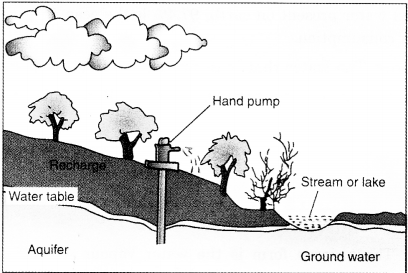We hope the NCERT Solutions for Class 7 Science Chapter 16 Water: A Precious Resource help you. If you have any query regarding NCERT Solutions for Class 7 Science Chapter 16 Water: A Precious Resource, drop a comment below and we will get back to you at the earliest.

## NCERT Solutions for Class 7 Maths Chapter 8 Comparing Quantities Ex 8.1

NCERT Solutions for Class 7 Maths Chapter 8 Comparing Quantities Ex 8.1 are part of NCERT Solutions for Class 7 Maths. Here we have given NCERT Solutions for Class 7 Maths Chapter 8 Comparing Quantities Ex 8.1.

 Board CBSE Textbook NCERT Class Class 7 Subject Maths Chapter Chapter 8 Chapter Name Comparing Quantities Exercise Ex 8.1 Number of Questions Solved 3 Category NCERT Solutions

## NCERT Solutions for Class 7 Maths Chapter 8 Comparing Quantities Ex 8.1

Question 1.
Find the ratio of:
(a) ₹ 5 to 50 paise
(b) 15 kg to 210 g
(c) 9 m to 27 cm
(d) 30 days to 36 hour

Solution:
(a) ₹ 5 : 50 paise
= ₹ 5 : 50 paise
= 5 × 100 paise : 50 paise
= 500 paise : 50 paise
= 10 : 1.

(b) 15 kg to 210g
= 15 kg : 210g
= 15 × 1000 g : 210g
= 15000 g : 210g = 500:7.

(c) 9 m to 27 cm
= 9m : 27 cm = 9 × 100 cm : 27 cm
= 900 cm : 27 cm
900 : 27 = 100 : 3.

(d) 30 days to 36 hours
= 30 days : 36 hours
30 × 24 hours : 36 hours
= 720 hours : 36 hours
= 720 : 36 = 20 : 1.

Question 2.
In a computer lab, there are 3 computers for every 6 students. How many computers will be needed for 24 students?
Solution:
Let x computers be needed for 24 students.Hence, 12 computers will be needed for
24 students.
Aliter:
In a computer lab,
Every 6 students need 3 computers.
∴ Every 1 student needs $$\frac { 3 }{ 6 }$$ computers.
∴ Every 24 students need $$\frac { 3 }{ 6 }$$ × 24 = 3 × 4 = 12 computers

Question 3.
Population of Rajasthan = 570 lakhs
and population of UP = 1660 lakhs.
Area of Rajasthan =3 lakh km2
and area of UP = 2 lakh km2.
(i) How many people are there per km2 in both these States?
(ii) Which State is less populated?
Solution:
(i) Number of people per sq. km. in Rajasthan state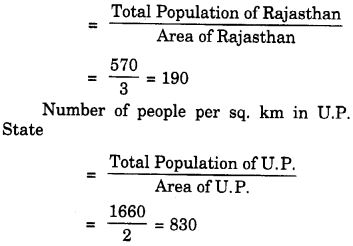(ii) The Rajasthan State is less populated.

We hope the NCERT Solutions for Class 7 Maths Chapter 8 Comparing Quantities Ex 8.1 helps you. If you have any query regarding NCERT Solutions for Class 7 Maths Chapter 8 Comparing Quantities Ex 8.1, drop a comment below and we will get back to you at the earliest.

## NCERT Solutions for Class 7 Science Chapter 10 Respiration in Organisms

NCERT Solutions for Class 7 Science Chapter 10 Respiration in Organisms are part of NCERT Solutions for Class 7 Science. Here we have given NCERT Solutions for Class 7 Science Chapter 10 Respiration in Organisms.

 Board CBSE Textbook NCERT Class Class 7 Subject Science Chapter Chapter 10 Chapter Name Respiration in Organisms Number of Questions Solved 9 Category NCERT Solutions

## NCERT Solutions for Class 7 Science Chapter 10 Respiration in Organisms

Question 1.
Why does an athlete breathe faster and deeper than usual after finishing the race?
During running, the athlete uses a lot of energy. So, he/she needs more energy and breathes faster.

Question 2.
List the similarities and differences between aerobic and anaerobic respiration.
Similarities:

 Aerobic Respiration Anaerobic Respiration 1. It starts with the breakdown of a nutrient (glucose). 1. It also starts with the breakdown of a nutrient (glucose). 2. It yields byproducts. 2. It also yields byproducts. 3. It takes place in a cell. 3. It also takes place in a cell. 4. In this process energy is released. 4. In this process also energy is released.

Differences:

 Aerobic Respiration Anaerobic Respiration 1. It is the process of breakdown of glucose in the presence of oxygen. 1. It is the process of breakdown of glucose in the absence of oxygen. 2. Glucose is completely oxidized. 2. Glucose is incompletely oxidized. 3. The end products formed are CO2, H2O, and energy. 3. The end products formed are C02, ethyl alcohol (organic acid), and energy. 4. Energy released is more. (38 ATP molecules). 4. Energy released is less (2 ATP molecules). 5. It takes place in all higher organisms. 5. It takes place in lower organisms like yeast and the muscles of man. 6. The reactions take place in the cytoplasm and mitochondria. 6. The reactions take place only in the cytoplasm.

Question 3.
Why do we often sneeze when we inhale a lot of dust-laden air?
The air around us has various types of unwanted particles, such as smoke, dust, pollens, etc. When we inhale, the particles get trapped in the hair present in our nasal cavity. However, sometimes these particles may get past the hair in thç nasal cavity. Then they irritate the lining of the cavity, as a result of which we sneeze. Sneezing expels these foreign particles from the inhaled air and dust-free, clean air enters our body.

Question 4.
Take three test-tubes. Fill 3/4th of each with water. Label them A, B and C. Keep a snail in test-tube A, a water plant in test-tube B, and in C keep snail and plant both. Which test-tube would have the highest concentration of CO2?
The exchange of gases in three test-tubes can be shown as in Fig.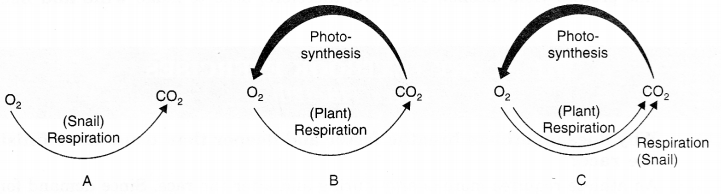Fig. (A) In test tube A; CO2 is produced by the respiration of snail (No consumption of CO2).
(B) In test tube B; CO2 is produced by the respiration of the plant and a much higher amount of CO2 is consumed during photosynthesis.
(C) In test tube C; CO2 is produced by the respiration of snail and plant and CO2 is consumed during photosynthesis.
It is clear from the above observation that the highest concentration of CO2 will be in test tube A.

Question 5.
(a) In cockroaches, air enters the body through
(i) lungs
(ii) gills
(iii) spiracles
(iv) skin

(b) During heavy exercise, we get cramps in the legs due to the accumulation of
(i) carbon dioxide
(ii) lactic acid
(iii) alcohol
(iv) water

(c) Normal range of breathing rate per minute in an average adult person at rest is:
(i) 9-12
(ii) 15-18
(iii) 21-24
(iv) 30-33

(d) During exhalation, the ribs
(i) move outwards
(ii) move downwards
(iii) move upwards
(iv) do not move at all
(a) (iii) spiracles
(b) (ii) lactic acid
(c) (ii) 15 – 18
(d) (ii) move downwards

Question 6.
Match the items in Column I with those in Column II :

 Column I Column II (a) Yeast (i) Earthworm (b) Diaphragm (ii) Gills (c) Skin (iii) Alcohol (d) Leaves (iv) Chest cavity (e) Fish (v) Stomata (f) Frog (vi) Lungs and skin (vii) Tracheae

 Column I Column II (a) Yeast (iii) Alcohol (b) Diaphragm (iv) Chest cavity (c) Skin (i) Earthworm (d) Leaves (v) Stomata (e) Fish (ii) Gills (f) Frog (vi) Lungs and skin

Question 7.
Mark ‘T’ if the statement is true and ‘F’ if it is false :
During heavy exercise, the breathing rate of a person slows down. (T/F)
Plants carry out photosynthesis only during the day and respiration only at night. (T/F)
Frogs breathe through their skins as well as their lungs. (T/F)
The fishes have lungs for respiration. (T/F)
The size of the chest cavity increases during inhalation. (T/F)
(i) F
(ii) F
(iii) T
(iv) F
(v) T

Question 8.
Given below is a square of letters in which are hidden different words related to respiration in organisms. These words may be present in any direction—upwards, downwards, or along the diagonals. Find the words for your respiratory system. Clues about those words are given below the square.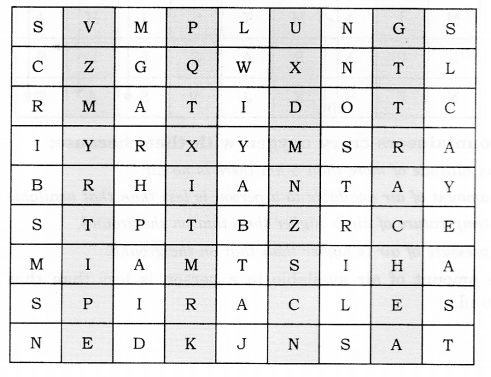(i) The air tubes of insects
(ii) Skeletal structures surrounding the chest cavity
(iii) Muscular floor of the chest cavity
(iv) Tiny pores on the surface of the leaf
(v) Small openings on the sides of the body of an insect
(vi) The respiratory organs of human beings
(vii) The openings through which we inhale
(viii) An anaerobic organism
(ix) An organism with a tracheal system
(i) TRACHEA
(ii) RIBS
(iii) DIAPHRAGM
(iv) STOMATA
(v) SPIRACLES
(vi) LUNGS
(vii) NOSTRILS
(viii) YEAST
(ix) ANT
These names are indicated by arrows and their serial number are indicated at starting point of the arrow.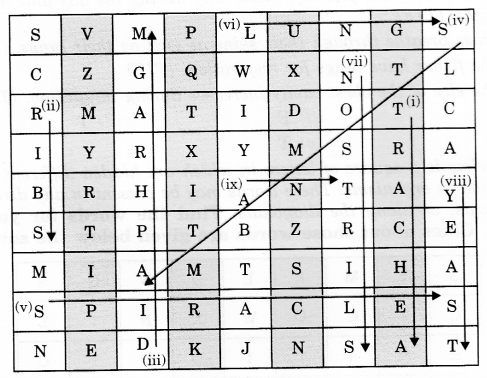Question 9.
The mountaineers carry oxygen with them because:
(a) at an altitude of more than 5 km, there is no air.
(b) the amount of air available to a person is less than that available on the ground.
(c) the temperature of the air is higher than that on the ground.
(d) the pressure of air is higher than that on the ground.
(b) The amount of air available to a person is less than that available on the ground.

We hope the NCERT Solutions for Class 7 Science Chapter 10 Respiration in Organisms help you. If you have any query regarding NCERT Solutions for Class 7 Science Chapter 10 Respiration in Organisms, drop a comment below and we will get back to you at the earliest.

## NCERT Solutions for Class 7 Science Chapter 15 Light

NCERT Solutions for Class 7 Science Chapter 15 Light are part of NCERT Solutions for Class 7 Science. Here we have given NCERT Solutions for Class 7 Science Chapter 15 Light.

 Board CBSE Textbook NCERT Class Class 7 Subject Science Chapter Chapter 15 Chapter Name Light Number of Questions Solved 13 Category NCERT Solutions

## NCERT Solutions for Class 7 Science Chapter 15 Light

Question 1.
Fill in the blanks:

1. An image that cannot be obtained on a screen is called ………
2. The image formed by a convex ……… is always virtual and smaller in size.
3. An image formed by a ………. mirror is always of the same size as that of the object.
4. An image which can be obtained on a screen is called a ………. image.
5. An image formed by a concave ………. cannot be obtained on a screen.

1. virtual image
2. mirror
3. plane
4. real
5. lens

Question 2.
Mark “T” if the statement is true and ‘F’ if it is false:

1. We can obtain an enlarged and erect image by a convex mirror. (T/F)
2. A concave lens always forms a virtual image. (T/F)
3. We can obtain a real, enlarged, and inverted image by a concave mirror. (T/F)
4. A real image cannot be obtained on a screen. (T/F)
5. A concave mirror always forms a real image. (T/F)

1. F
2. T
3. T
4. F
5. F

Question 3.
Match the items given in Column I with one or more items of Column II.

 Column I Column II (a) A plane mirror (i) Used as a magnifying glass. (b) A convex mirror (ii) Can form images of objects spread over a large area. (c) A convex lens (iii) Used by dentists to see an enlarged images of teeth. (d) A concave mirror (iv) The image is always inverted and magnified. (e) A concave lens (v) The image is erect and of the same size as the object. (vi) The image is erect and smaller in size than the object.

 Column I Column II (a) A plane mirror (v) The image is erect and of the same size as the object. (b) A convex mirror (ii) Can form images of objects spread over a large area. (c) A convex lens (i) Used as a magnifying glass. (d) A concave mirror (iii) Used by dentists to see enlarged images of teeth. (e) A concave lens (vi) The image is erect and smaller in size than the object.

Question 4.
State the characteristics of the image formed by a plane mirror.
Image formation by a plane mirror. We are able to see images using a mirror. An image formed by a mirror (flat) has the following features: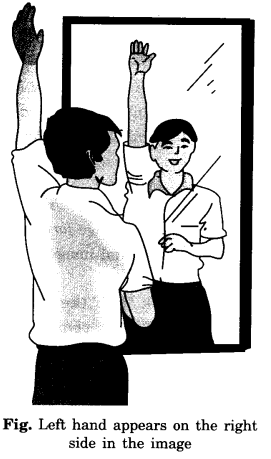In a plane mirror, the image is formed behind the mirror. It is erect, of the same size, and is at the same distance from the mirror as the object is in front of it.

Question 5.
Find out the letters of the English alphabet or any other language known to you in which the image formed in a plane mirror appears exactly like the letter itself. Discuss your findings.
A, H, I, M, 0, T, U, V, W, X, Y.

Question 6.
What is a virtual image? Give one situation where a virtual image is formed.
The image that cannot be formed on a screen is called a virtual image. The image formed by a plane mirror is virtual because the image cannot be obtained on a screen when placed either in front of the mirror or behind it.

Question 7.
State two differences between a convex and a concave lens.

 Convex lens Concave lens 1. It is thicker in the middle and thinner at the edges. 1. It is thinner in the middle and thicker at the edges. 2. Converges the light falling on it. 2. Diverges the light falling on it. 3. Can form virtual, erect, and magnified images. 3. Always forms erect, virtual, and smaller images.

Question 8.
Give one use each of a concave and a convex mirror.

1. Concave mirror: Used by dentists to see teeth.
2. Convex mirror: Used in vehicles as a rearview mirror.

Question 9.
Which type of mirror can form a real image?
A concave mirror can form a real image.Fig. Convex mirror as a side-view mirror

Question 10.
Which type of lens forms always a virtual image?
A concave lens.

Choose the correct option in questions 11-13:

Question 11.
A virtual image larger than the object can be produced by a
(i) concave lens
(ii) concave mirror
(iii) convex mirror
(iv) plane mirror
(ii) Concave mirror

Question 12.
David is observing his image in a plane mirror. The distance between the mirror and his image is 4 m. If he moves 1 m towards the mirror, then the distance between David and his image will be:
(i) 3 m
(ii) 5 m
(iii) 6 m
(iv) 8 m
(iii) 6 m

Question 13.
The rearview mirror of a car is a plane mirror. A driver is reversing his car at a speed of 2 m/s. The driver sees in his rearview mirror the image of a truck parked behind his car. The speed at which the image of the truck appears to approach the driver will be:
(i) 1 m/s
(ii) 2 m/s
(iii) 4 m/s
(iv) 8 m/s
(iii) 4 m/s

We hope the NCERT Solutions for Class 7 Science Chapter 15 Light helps you. If you have any query regarding NCERT Solutions for Class 7 Science Chapter 15 Light, drop a comment below and we will get back to you at the earliest.

## NCERT Solutions for Class 7 Science Chapter 14 Electric Current and its Effects

NCERT Solutions for Class 7 Science Chapter 14 Electric Current and its Effects are part of NCERT Solutions for Class 7 Science. Here we have given NCERT Solutions for Class 7 Science Chapter 14 Electric Current and its Effects.

 Board CBSE Textbook NCERT Class Class 7 Subject Science Chapter Chapter 14 Chapter Name Electric Current and its Effects Number of Questions Solved 13 Category NCERT Solutions

## NCERT Solutions for Class 7 Science Chapter 14 Electric Current and its Effects

Question 1.
Draw in your notebook the symbols to represent the following components of electrical circuits: connecting wires, switch in the ‘OFF’ position, bulb, cell, switch in the ‘ON’ position and battery.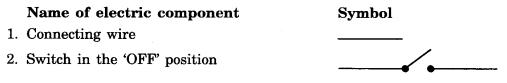Question 2.
Draw the circuit diagram to represent the circuit shown in Fig.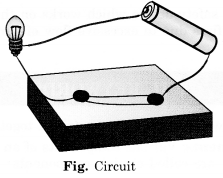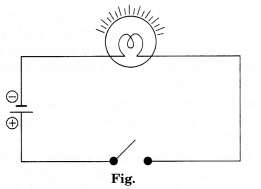Question 3.
Figure shows four cells fixed on a board. Draw lines to indicate how you will connect their terminals with wires to make a battery of four cells.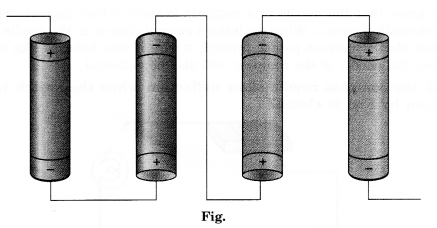Question 4.
The bulb in the circuit shown in Fig. does not glow. Can you identify the problem? Make necessary changes in the circuit to make the bulb glow.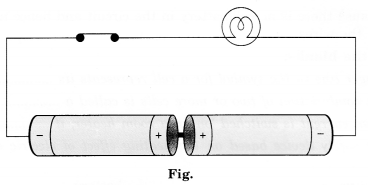The problem in this circuit is the connection of two cells. Here both the positive terminals of the cells are connected to each other. This must be reversed for anyone cell to make the bulb glow i.e., the positive terminal of one cell should be connected with the negative terminal of the other.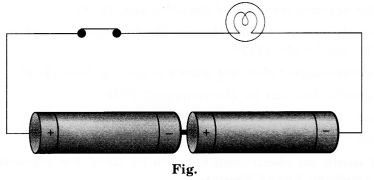Question 5.
Name any two effects of electric current.

• Heating effect.
• Magnetic effect.

Question 6.
When the current is switched on through a wire, a compass needle kept nearby gets deflected from its north-south position. Explain.
When an electric current passes through a wire, it behaves like a magnet. This is the magnetic effect of the electric current due to the attraction of the wire. So, the compass needle which is a magnet gets deflected.

Question 7.
Will the compass needle show deflection when the switch in the circuit shown by Fig. is closed?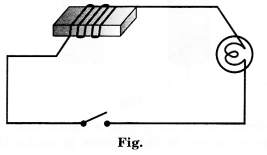No. Since, there is no cell so, no current will flow.

Question 8.
Fill in the blanks:

1. A longer line in the symbol for a cell represents its ………… terminal.
2. The combination of two or more cells is called a …………..
3. When current is switched ‘on’ in a room heater, it ………….
4. The safety device based on the heating effect of electric current is called a ……….

1. positive battery
2. battery
3. becomes red hot
4. fuse

Question 9.
Mark ‘T’ if the statement is true and ‘F’ if it is false:

1. To make a battery of two cells, the negative terminal of one cell is connected to the negative terminal of the other cell. (T/F)
2. When the electric current, through the fuse exceeds a certain limit, the fuse wire melts and breaks. (T/F)
3. An electromagnet does not attract a piece of iron. (T/F)
4. An electric bell has an electromagnet. (T/F)

1. False
2. True
3. False
4. True

Question 10.
Do you think an electromagnet can be used for separating plastic bags from a garbage heap? Explain.
No. This is because electromagnets can only attract magnetic materials. The plastic bag is a non-magnetic material and will not be attracted by an electromagnet.

Question 11.
An electrician is carrying out some repairs in your house. He wants to replace a fuse with a piece of wire. Would you agree? Give reasons for your response.
No, we would not agree to replace the fuse with a wire. The electric wire will not melt even if a high current flows through it. So, it will not prevent the damage done by high current.

Question 12.
Zubeda made an electric circuit using a cell holder shown in the following Fig., a switch, and a bulb. When she put the switch in the ‘ON’ position, the bulb did not glow. Help Zubeda in identifying the possible defects in the circuit.One of the reasons may be that the rubber band used in the cell holder may not be tight enough to keep the two cells in contact with each other. If the cells are not in proper contact with each other, then the circuit will not be complete, and the current will not flow through the circuit. Hence, the bulb will not glow. The other reason may be that the two cells are not connected properly. The negative terminal of one cell must be connected to the positive terminal of the other cell.

Question 13.
In the circuit shown in the following Fig.: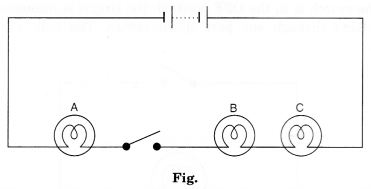1. Would any of the bulbs glow when the switch is in the ‘OFF’ position?
2. What will be the order in which the bulbs A, B, and C will glow when the switch is moved to the ‘ON’ position?

1. No bulb will glow
2. All bulbs will glow at a time.

We hope the NCERT Solutions for Class 7 Science Chapter 14 Electric Current and its Effects help you. If you have any query regarding NCERT Solutions for Class 7 Science Chapter 14 Electric Current and its Effects, drop a comment below and we will get back to you at the earliest.

## NCERT Solutions for Class 7 Maths Chapter 6 The Triangle and its Properties Ex 6.1

NCERT Solutions for Class 7 Maths Chapter 6 The Triangle and its Properties Ex 6.1 are part of NCERT Solutions for Class 7 Maths. Here we have given NCERT Solutions for Class 7 Maths Chapter 6 The Triangle and its Properties Ex 6.1.

 Board CBSE Textbook NCERT Class Class 7 Subject Maths Chapter Chapter 6 Chapter Name The Triangle and its Properties Exercise Ex 6.1 Number of Questions Solved 3 Category NCERT Solutions

## NCERT Solutions for Class 7 Maths Chapter 6 The Triangle and its Properties Ex 6.1

Question 1.
In ∆PQR, D is the mid-point of $$\overline { QR }$$.Solution:
$$\overline { PM }$$ is the altitude.
PD is the median.
No! QM ≠ MR.

Question 2.
Draw rough sketches for the following:

(a) In ∆ ABC, BE is a median.
(b) In ∆ PQR, PQ and PR are altitudes of the triangle.
(c) In ∆ XYZ, YL is an altitude in the exterior of the triangle.

Solution: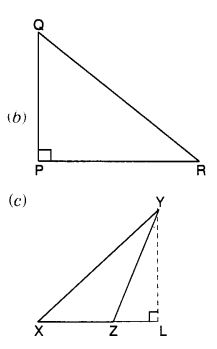Question 3.
Verify by drawing a diagram if the median and altitude of an isosceles triangle can be the same.
Solution:
AL is the altitude.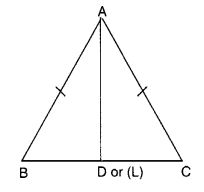Draw a line segment BC. By paper folding, locate the perpendicular bisector of BC. The folded crease meets BC at D, its mid-point.
Take any point A on this perpendicular bisector. Join AB and AC. The triangle thus obtained is an isosceles ∆ABC in which AB = AC.
Since D is the mid-point of BC, so AD is its median. Also, AD is the perpendicular bisector of BC. So, AD is the altitude of ∆ABC.
Thus, it is verified that the median and altitude of an isosceles triangle are the same.

We hope the NCERT Solutions for Class 7 Maths Chapter 6 The Triangle and it’s Properties Ex 6.1 help you. If you have any query regarding NCERT Solutions for Class 7 Maths Chapter 6 The Triangle and its Properties Ex 6.1, drop a comment below and we will get back to you at the earliest.

## NCERT Solutions for Class 7 Maths Chapter 12 Algebraic Expressions Ex 12.1

NCERT Solutions for Class 7 Maths Chapter 12 Algebraic Expressions Ex 12.1 are part of NCERT Solutions for Class 7 Maths. Here we have given NCERT Solutions for Class 7 Maths Chapter 12 Algebraic Expressions Ex 12.1.

 Board CBSE Textbook NCERT Class Class 7 Subject Maths Chapter Chapter 12 Chapter Name Algebraic Expressions Exercise Ex 12.1 Number of Questions Solved 7 Category NCERT Solutions

## NCERT Solutions for Class 7 Maths Chapter 12 Algebraic Expressions Ex 12.1

Question 1.
Get the algebraic expressions in the following cases using variables, constants, and arithmetic operations.
(i) Subtraction of z from y.
Solution:
y – z

(ii) One-half of the sum of numbers x and y.
Solution:
$$\frac{1}{2}$$  (x -y)

(iii) The number z multiplied by itself.
Solution:
z × z i.e., z2

(iv) One-fourth of the product of numbers p and q.
Solution:
$$\frac{1}{4}$$ pq

(v) Numbers x and y both squared and added.
Solution:
x2 + y2

(vi) Number 5 added to three times the product of numbers m and n.
Solution:
3mn + 5

(vii) Product of numbers y and z subtracted from 10.
Solution:
10 – yz

(viii) Sum of numbers a and 6 subtracted from their product.
Solution:
ab – (a + b)

Question 2.
(i) Identify the terms and their factors in the following expressions. Show the terms and factors by tree diagrams :

(a) x – 3
(b) 1 + x + x2
(c) y – y3
(d) 5xy2 + 7x2y
(e) -ab + 2b2 -3a2

(ii) Identify terms and factors in the expressions given below :

(a) – 4x + 5
(b) – 4x + 5y
(c) 5y + 3y2
(d) xy + 2x2y2
(e) pq + q
(f) 1.2 ab -2.4 b + 3.6 a
(g) $$\frac { 3 }{ 4 }$$ x + $$\frac { 1 }{ 4 }$$
(h) 0.1 p2 + 0.2 q2

Solution: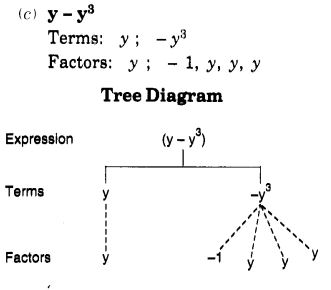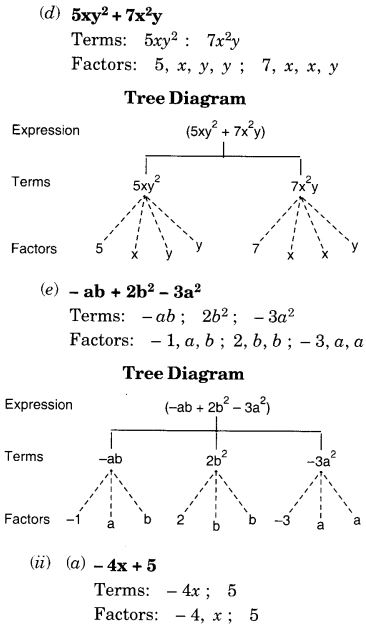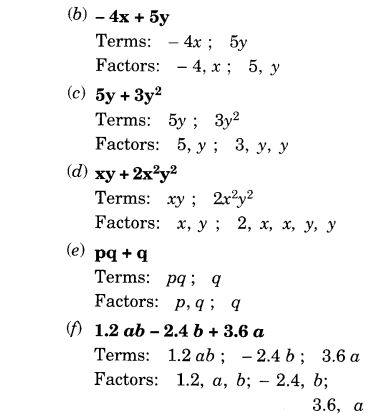Question 3.
Identify the numerical coefficients of terms (other than constants) in the following expressions :

1. 5 – 3t2
2. 1 + t + t2 + t3
3. x + 2xy + 3y
4. 100m + 1000n
5. -p2q2 + 7pq
6. 1.2 a + 0.8 b
7. 3.14 r2
8. 2 (l + b)
9. 0.1 y + 0.01 y2.

Solution: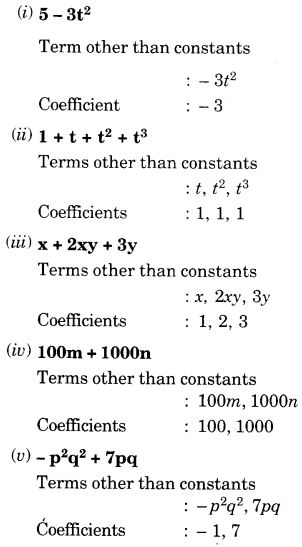Question 4.
(a) Identify terms which contain x and give the coefficient of x.

1. y2x + y
2. 13y2 – 8yx
3. x + y + 2
4. 5 + z + zx
5. 1 + x + xy
6. 12xy2 + 25
7. 7x + xy2.

(b) Identify terms which contain y2 and give the coefficient of y2.

1. 8 – xy2
2. 5y2 + 7x
3. 2x2y – 15xy2 + 7y2

Solution: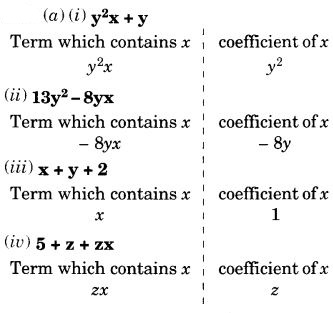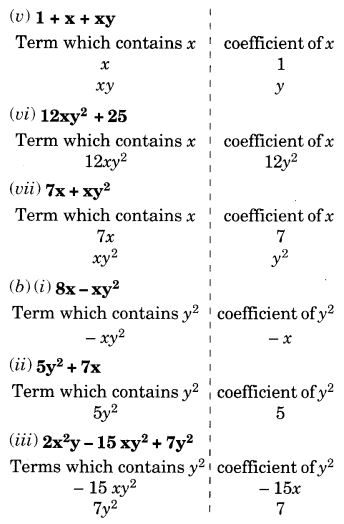Question 5.
Classify into monomials, binomials and trinomials.

1. 4y – 7z
2. y2
3. x + y – xy
4. 100
5. ab – a – b
6. 5 – 3t
7. 4p2q – 4pq2
8. 7mn
9. z2 – 3z + 8 a2 + b2
10. z2 + z
11. 1 + x+ x2

Solution:
We know that an algebraic expression containing only one term is called a monomial. So, the monomials are : (ii), (iv), and (viii).

We know that an algebraic expression containing two terms is called a binomial. So, the binomials are : (i), (vi), (vii), (x) and (xi).

We know that an algebraic expression containing three terms is called a trinomial. So, the trinomial are : (iii), (v), (ix) and (xii).

Question 6.
State whether a given pair of terms is of like or unlike terms :
(i) 1, 100
Solution:
Like

(ii) -7x, $$\frac{5}{2}$$x
Solution:
Like

(iii) – 29x, – 29y
Solution:
Unlike

(iv)14xy, 42yx
Solution:
Like

(v) 4m2p, 4mp2
Unlike

(vi) 12xz, 12x2z2
Solution:
Unlike

(i) 4y – 7z.
This expression is a binomial because it contains two terms: 4y and – Iz.
(ii) y2.
This expression is a monomial because it contains only one term: y2
(iii) x + y – xy.
This expression is a trinomial because it contains three terms: x, y, and – xy.
(iv) 100.
This expression is a monomial because it contains only one term: 100
(v) ab – a – b.
This expression is a trinomial because it contains three terms: ab, -a, and -b
(vi) 5 – 3t.
This expression is a binomial because it contains two terms : 5 and – 31.
(vii) 4p2q – 4pq2.
This expression is a binomial because it contains two terms: 4p2q and – 4pq2.
(viii) 7mn.
This expression is a monomial because it contains only one term : 7mn.
(ix) z2 – 3z + 8.
This expression is a trinomial because it contains three terms : z2, – 3z and 8.
(x) a2 + b2.
This expression is a binomial because it contains two terms: a2 and b2.
(xi) z2 + z.
This expression is a binomial because it contains two terms : z2 and z.
(xii) 1 + x + x2.
This expression is a trinomial because it contains three terms: 1, x, and x2.

Question 6.
State whether a given pair of terms is of like or unlike terms :
(i) 1, 100
Solution:
Like

(ii) -7x, $$\frac{5}{2}$$x
Solution:
Like

(iii) – 29x, – 29y
Solution:
Unlike

(iv)14xy, 42yx
Solution:
Like

(v) 4m2p, 4mp2
Unlike

(vi) 12xz, 12x2z2
Solution:
Unlike

Question 7.
Identify like terms in the following :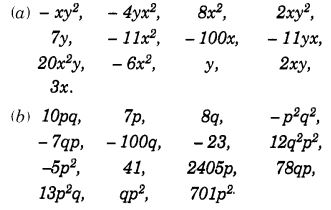Solution: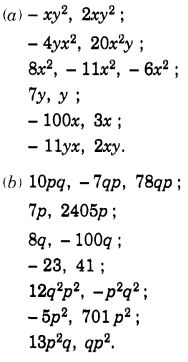We hope the NCERT Solutions for Class 7 Maths Chapter 12 Algebraic Expressions Ex 12.1 help you. If you have any query regarding NCERT Solutions for Class 7 Maths Chapter 12 Algebraic Expressions Ex 12.1, drop a comment below and we will get back to you at the earliest.

## RS Aggarwal Class 7 Solutions Chapter 17 Constructions Ex 17B

These Solutions are part of RS Aggarwal Solutions Class 7. Here we have given RS Aggarwal Solutions Class 7 Chapter 17 Constructions Ex 17B.

Other Exercises

Question 1.
Solution:
Steps of construction :
(i) Draw a line segment BC = 3.6cm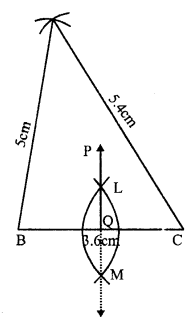(ii) At B, draw an arc of the radius 5cm.
(iii) At C, draw another arc of the radius 5.4cm which intersects the first arc at A
(iv) Join AB and AC
(v) With centre B and C and radius more than half of BC, draw arcs intersecting each other at L and M.
(vi) Join LM which intersects BC at Q and produce it to P.
Then PQ is perpendicular bisector of side BC.

Question 2.
Solution:
Steps of construction :
(i) Draw a line segment QR = 6cm
(ii) With centre Q and radius 4.4 cm draw an arc.
(iii) With centre R and radius 5.3 cm, draw another arc intersecting the first arc at P.
(iv) Join PQ and PR.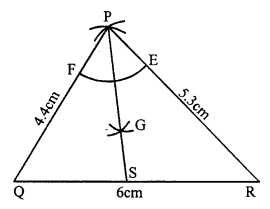(v) With centre P and a suitable radius, draw an arc meeting PR at E and PQ at F.
(vi) With centres E and F, with same radius, draw two arcs intersecting each other at G.
(vii) Join PG and produce it to meet QR at S. Then PS is the bisector of ∠P.

Question 3.
Solution:
Steps of construction :
(i) Draw a line segment BC = 6.2 cm.
(ii) With centres B and C radius 6.2 cm, draw arcs intersecting each other at A.
(iii) Join AB and AC.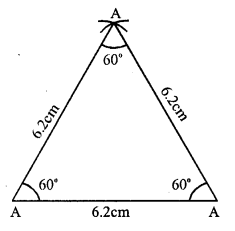∆ABC is the required equilateral triangle.
On measuring, each angle is equal to 60°.

Question 4.
Solution:
Steps of construction :
(i) Draw a line segment BC = 5.3 cm.
(ii) With centre B and C, and radius 4.8 cm, draw arcs intersecting each other at A
(iii) Join AB and AC, Then ∆ABC is the required triangle.
(iv) Now, with centre A and a suitable radius draw an arc intersecting BC at L and M.
(v) Then with centre L and M, draw two arcs intersecting eachother at E.
(vi) Join AE intersecting BC at D. Then AD is perpendicular to BC.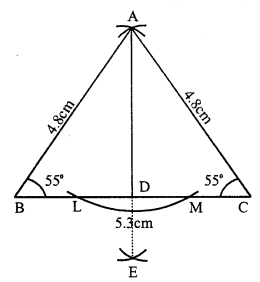On measuring ∠B and ∠C, each is equal to 55°.

Question 5.
Solution:
Steps of construction :
(i) Draw a line segment AB = 3.8cm.
(ii) At A draw a ray AX making an angle of 60°.(iii) Cut off AC = 5 cm from AX
(iv) Join CB.
Then ∆ABC is the required triangle.

Question 6.
Solution:
Steps of construction :
(i) Draw a line segment BC = 4.3 cm.
(ii) At C, draw a ray CY making an angle equal to 45°.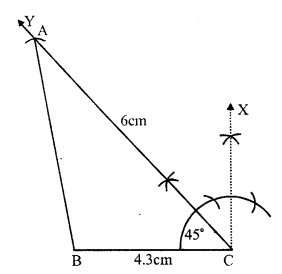(iii) With centre C, and radius 6cm, draw an arc intersecting CY at A.
(iv) Join AB.
Then ∆ABC is the required triangle.

Question 7.
Solution:
(i) Draw a line segment AB = 5.2cm.
(ii) At A, draw a ray AX making an angle equal to 120°.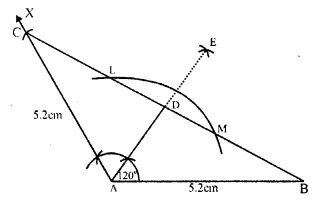(iii) From AX cut off AC = 5.2cm.
(iv) Join BC.
Then ∆ABC is the required triangle.
(v) With centre A and some suitable radius draw an arc intersecting BC at L and M.
(vi) With centres L and M, draw two arcs intersecting each other at E.
(vii) Join AE intersecting BC at D Then AD is the perpendicular to BC.

Question 8.
Solution:
Steps of construction :
(i) Draw a line segment BC = 6.2 cm.
(ii) At B draw a ray BX making an angle of 60°.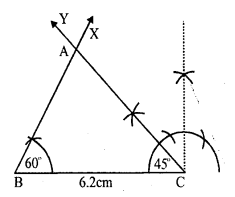(iii) At C draw another ray CY making an angle of 45° which intersect the ray BX at A. .
Then ∆ABC is the required triangle.

Question 9.
Solution:
Steps of construction :
(i) Draw a line segment BC = 5.8 cm.
(ii) At B draw a ray BX making an angle of 30°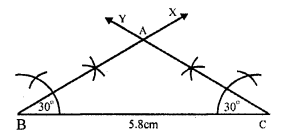(iii) At C draw another ray CY making an angle of 30° intersecting the BX at A
Then ∆ABC is the required triangle.
On measuring AB and AC, AB = 3.5cm and AC = 3.5cm.
AB = AC
∆ABC is an isosceles triangle.

Question 10.
Solution:
Steps of construction :
In ∆ABC, ∠A = 45° and ∠C = 75°
But ∠A + ∠B + ∠C = 180°⇒ 45° + ZB + 75° = 180°
⇒ ∠B = 180° – 45° – 75°
⇒ ∠B = 180° – 120° = 60°
(i) Draw a line segment AB = 7cm.
(ii) At A, draw a ray AX making an angle of 45°.
(iii) At B, draw another ray BY making an angle of 60° which intersects AX at C
Then ∆ABC is the required triangle.

Question 11.
Solution:
Steps of construction :
(i) Draw a line segment BC = 4.8 cm
(ii) At C, draw a ray CX making an angle of 90°.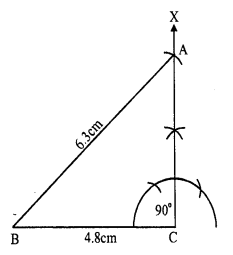(iii) With centre B, an radius 6.3cm draw an arc intersecting CX at A.
(iv) Join AB.
Then ∆ABC is the required triangle.

Question 12.
Solution:
Steps of construction :
(i) Draw a line segment BC = 3.5cm
(ii) At B, draw a ray BX making an angle of 90°.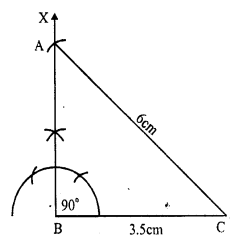(iii) With centre C and radius 6cm draw an arc intersecting BX at A
(iv) Join AC.
Then ∆ABC is the required triangle.

Question 13.
Solution:
One acute angle = 30°, then
second acute angle will be = 90° – 30° = 60° (Sum of acute angles = 90°)
Steps of construction :
(i) Draw a line segment BC = 6cm.
(ii) At B draw a ray BX making an angle of 30°.
(iii) At C draw another ray CY making an angle of 60° which intersects BX at A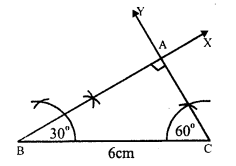Then ∆ABC is the required triangle.

Hope given RS Aggarwal Solutions Class 7 Chapter 17 Constructions Ex 17B are helpful to complete your math homework.

If you have any doubts, please comment below. Learn Insta try to provide online math tutoring for you.

## NCERT Solutions for Class 7 English An Alien Hand Chapter 8 The Bear Story

NCERT Solutions for Class 7 English An Alien Hand Chapter 8 The Bear Story are part of NCERT Solutions for Class 7 English. Here we have given NCERT Solutions for Class 7 English An Alien Hand Chapter 8 The Bear Story.

 Board CBSE Textbook NCERT Class Class 7 Subject English An Alien Hand Chapter Chapter 8 Chapter Name The Bear Story Number of Questions Solved 8 Category NCERT Solutions

## NCERT Solutions for Class 7 English An Alien Hand Chapter 8 The Bear Story

EXERCISES
(Page 57)

Question 1.
Where did the lady find the bear cub ? How did she bring it up ?
The lady found the bear cub in the dense forest near her house. She brought it up on the bottle with the help of the cook.

Question 2.
The bear grew up but “he was a most amiable bear”. Give three examples to prove this. (Imp.)
The bear was a most amiable bear. He did not dream of harming anybody, man or beast. He looked amicably at the cattle grazing in the field but caused them no harm. Sometimes he went into the stable with his mistress. The three horses there did not mind it in the least. The children used to ride his back. More than once they had been found asleep between his two paws.

Question 3.
What did the bear eat ? There were two things he was not allowed to do. What were they ?
The bear ate the same food as the dogs and often out of the same plate. He ate bread, porridge, potatoes, cabbages and turnips. Like all bears he was a vegetarian and he liked fruit the most. He was not allowed to climb the apple tree and eat them. He was also not allowed to touch the beehive.

Question 4.
When was the bear tied up with a chain ? Why ?
Generally the bear was tied up with a chain only at night. But on Sundays the lady visited her sister who lived on the other side of the mountain lake. It was not safe to go with the bear through the forest. So on Sundays the bear was chained all day.

Question 5.
What happened one Sunday when the lady was going to her sister’s house ? What did the lady do ? What was the bear’s reaction ? (Imp.)
One Sunday the lady was going to her sister’s house. She heard the cracking of tree branches in the forest. She looked back and was very angry to see the bear following her. She told him in her hardest tone to go back. The bear did not seem to listen. Then the lady saw that the bear had even lost his new collar. She hit the bear with her parasol so hard that it broke into two. The bear stopped and opened its mouth several times as if wanting to say something. Then the bear turned round and went back. However, it stopped now and then to look back at the lady. Ultimately the lady lost sight of him.

Question 6.
Why was the bear looking sorry for himself in the evening ? Why did the cook get angry with her mistress ? (V. Imp.)
The bear was looking sorry for himself in the evening. The poor creature had been on chain all day. He did not like to be chained. He was waiting for the lady to come and release him. However, when the lady came, she began to scold the bear. She also threatened him with punishment. The cook heard it and rushed out from the kitchen. She got angry with her mistress because she loved the bear much. She knew that the bear had been as gentle as an angel. So she asked her mistress to bless him instead of scolding him.

EXERCISES
[Page 57)

Discuss the following topics in groups.
Question 1.
Most people keep dogs and cats as pets. Can you think of some unusual pets that people keep ?